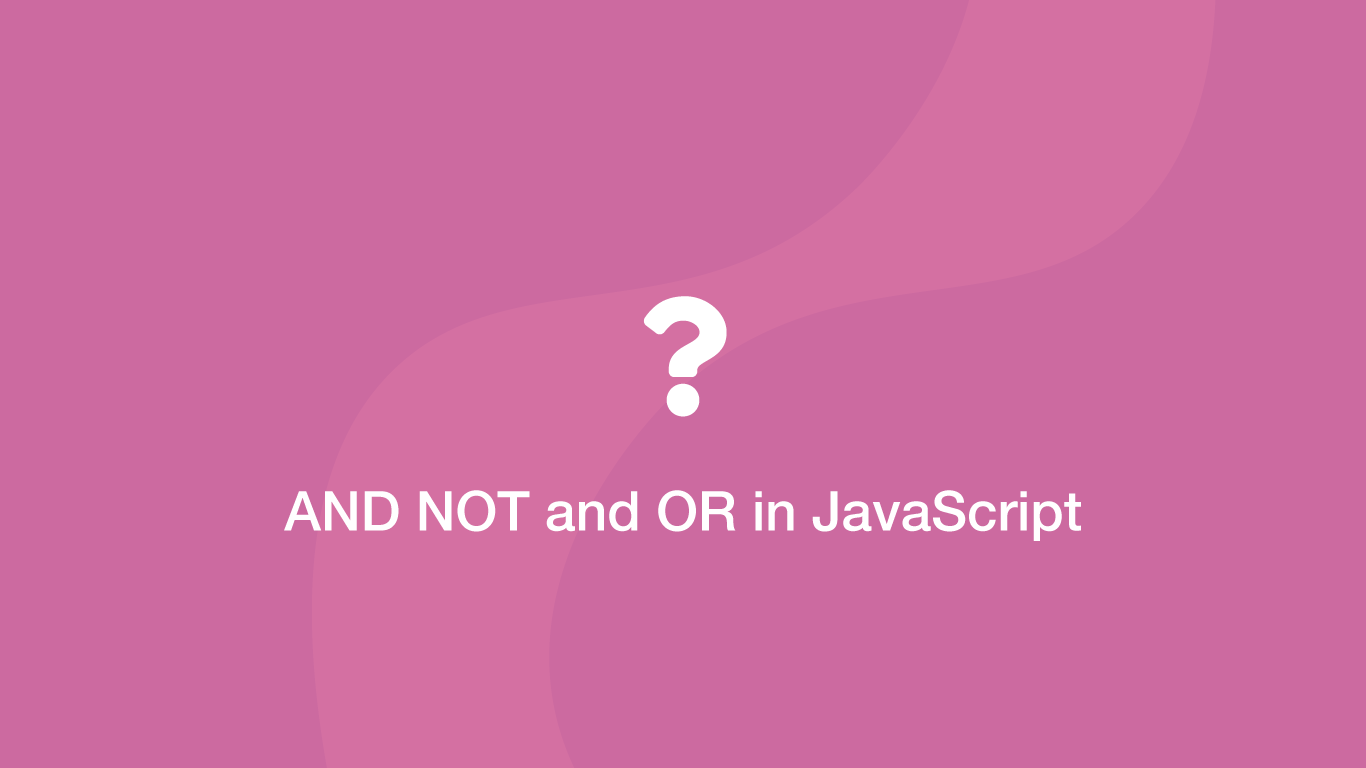# How to use AND, NOT and OR Logical Operators in JavaScriptIn JavaScript, we can evaluate the results from multiple comparison statements using the AND, NOT and OR logical operators. These operators will allow us to use as many value comparisons as needed inside a single statement, making the code more efficient.

In this tutorial, we will learn how to use the AND, OR and NOT operators in conjunction with the outcomes value comparisons.

## Logical OR Operator

In JavaScript, the OR operator is `||` (two pipes). Let's say we needed to check if at least one of two comparisons returns `true`. We could do this by putting an OR operator between them.

``````var result = 1 > 2 || 1 < 2;

console.log(result);
``````
``````true
``````

In the above example, we asking is 1 greater than 2 or is 1 less than 2. The first statement is `false` and the second is `true` so the `result` variable is `true`.

You can chain as many OR statements together as you need and as long as one of the comparisons is `true` you will get a `true` result back.

``````var result = 2 > 4 || 2 > 3 || 1 > 2 || 2 > 1;

console.log(result);
``````
``````true
``````

`2` is greater than `1` so the result variable is `true`.

## Logical AND Operator

The JavaScript AND operator is `&&` (two ampersands). The AND operator is used to check if all comparisons return `true`.

``````var result = 2 > 1 && 1 > 1;

console.log(result);
``````
``````false
``````

2 is greater than 1 and 1 is not greater than 1 so the `result` variable is `false`

## Logical NOT Operator

The JavaScript NOT operator is an `!` (exclamation mark). It is used to flip the logical `true` or `false` state of a result. Let's demonstrate how this works with an example.

``````var check = 1 == 1 || 2 == 2;

if (!check) {
console.log('run code here');
}
``````

The value of the `check` variable is `true` and inside the `if` statement we are flipping `true` to `false` using `!`. So the code in the `if` statement will only run if the value of `check` is `false`.

## Double Negative (!!) NOT Operator

The double not operator is `!!` (two exclamation marks) and will convert falsy or truthy values into boolean values.

``````console.log(!!false);
``````
``````false
``````
``````console.log(!!0);
``````
``````false
``````
``````console.log(!!"false");
``````
``````true
``````

## Conclusion

You now know how to perform multiple comparisons at once and when the OR or AND operators should be used. You also know that the NOT operator can flip a truthy or falsy result and a double negative will create a boolean value from a result.

#### Related Tutorials### How to Compare Values in JavaScript

August 26, 2020### How to Create and Use Arrays in JavaScript

July 11, 2020### How to Compare Strings in Python

September 20, 2020### How to Use Ternary Operators in JavaScript

September 09, 2020### The Modulus (Remainder) Operator in JavaScript

October 15, 2020### How to Copy to Clipboard with JavaScript

September 07, 2020